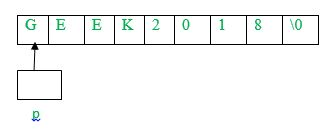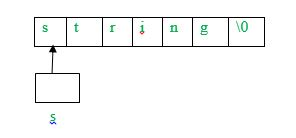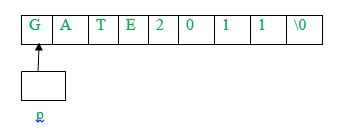Related Articles

# Practice questions on Strings

• Difficulty Level : Easy
• Last Updated : 21 Apr, 2020

String is an important topic from GATE exam point of view. We will discuss key points on strings as well different types of questions based on that.

There are two ways to store strings as character array (char p) or a pointer pointing to a string (char* s = “string”), both of which can be accessed as arrays. Assuming p as character array and s as pointer pointing to a string, following are the key points:

• The operator sizeof(p) will give the memory allocated to character array. However, sizeof(s) will give size of pointer which is independent of the string pointed by p.
• Each string is appended by a null character (‘\0’) which depicts end of the string.
• The length of string can be calculated using strlen() function. However, the function does not include null character ‘\0’ in the length. For example, strlen(s) will return 6.
• Single character from strings can be printed as:
```printf(“%c”, s);
printf(“%c”, p);```
• Complete string can be printed as:
```printf(“%s”, s);
printf(“%s”, p);```
• As p is a character array, its individual elements can be modified (p[i] = ‘c’). However, using s (a pointer to string), individual elements of string can’t be modified.
• As s is a pointer, it can be pointed to any other string as well (s =”string2”). However, using p, it is not possible.

Let us discuss some problems based on the concepts discussed:

Que – 1. What does the following fragment of C-program print?

```char c[] = "GEEK2018";
char *p =c;
printf("%c,%c", *p,*(p+p-p));```

(A) G, 1
(B) G, K
(C) GEEK2018
(D) None of the above

Solution: As given in the question, p points to character array c[] which can be represented as:As p is a pointer of type character, *p will print ‘G’

Using pointer arithmetic,
*(p+p-p) = *(p+75-69) (Using ascii values of K and E) = *(p+6) = 1(as we know p is holding the address of base string means 0th poision string, let assume the address of string starts with 2000 so p+6 means the address of p(we are adding 6 in 2000 that means 2006, and in 2006 the “1”is stored that is why answer is 1).
Therefore, the output will be G, 1.

Que – 2. Which of the following C code snippet is not valid?

(A) char* p = “string1”; printf(“%c”, *++p);
(B) char q[] = “string1”; printf(“%c”, *++q);
(C) char* r = “string1”; printf(“%c”, r);
(D) None of the above

Solution: Option (A) is valid as p is a pointer pointing to character ‘s’ of “string1”. Using ++p, p will point to character ‘t’ in “string1”. Therefore, *++p will print ‘t’.
Option (B) is invalid as q being base address of character array, ++q(increasing base address) is invalid.
Option (C) is valid as r is a pointer pointing to character ‘s’ of “string1”. Therefore,

`r = *(r+1) = ‘t’ and it will print ‘t’.`

Que – 3. Consider the following C program segment: (GATE CS 2004)

```char p;
char *s = "string";
int length = strlen(s);
int i;
for (i = 0; i < length; i++)
p[i] = s[length — i];
printf("%s",p);```

The output of the program is:
(A) gnirts
(B) gnirt
(C) string
(D) no output is printed

Solution: In the given code, p is declared as a character array and s is a pointer pointing to a string. The length will be initialized to 6. In the first iteration of for loop (i = 0),p[i] = s[6-0] and s is ‘\0’
Therefore, p becomes ‘\0’. As discussed, ‘\0’ means end of string. Therefore, nothing is printed as first character of string is ‘\0’.

Que – 4. What does the following fragment of C-program print?

```char c[] = "GATE2011";
char *p =c;
printf("%s", p + p - p) ;```

(A) GATE2011
(B) E2011
(C) 2011
(D) 011

Solution: As given in the question, p points to character array c[] which can be represented as:As p is a pointer of type character, using pointer arithmetic,
p + p – p = p + 69 – 65 (Using Ascii values of A and E) = p + 4

Now, p + 4 will point to 2, the string starting from 2 till ‘\0’ will be printed which is 2011.

Attention reader! Don’t stop learning now.  Practice GATE exam well before the actual exam with the subject-wise and overall quizzes available in GATE Test Series Course.

Learn all GATE CS concepts with Free Live Classes on our youtube channel.

My Personal Notes arrow_drop_up